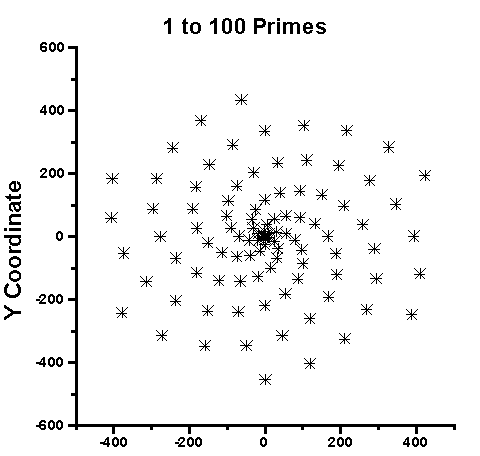Prime Number Theory

What if prime numbers were the only true numbers? What if chaos meant only that which humankind has not yet understood? What if prime number theory revealed the secret order underpinning everything? Interested in prime number theory, as a part of Chitchatuk life?

Read the article "Quantum-like Chaos in Prime Number Distribution and in Turbulent Fluid Flows" by A.M. Selvam here

Abstract

"Recent studies indicate a close association between the distribution of prime numbers and quantum mechanical laws governing the subatomic dynamics of quantum systems such as the electron or the photon. Number theoretical concepts are intrinsically related to the quantitative description of dynamical systems of all scales ranging from the microscopic subatomic dynamics to macroscale turbulent fluid flows such as the atmospheric flows. It is now recognised that Cantorian fractal spacetime characterise all dynamical systems in nature. A cell dynamical system model developed by the author shows that the continuum dynamics of turbulent fluid flows consist of a broadband continuum spectrum of eddies which follow quantumlike mechanical laws. The model concepts enable to show that the continuum real number field contains unique structures, namely prime numbers which are analogous to the dominant eddies in the eddy continuum in turbulent fluid flows. In this paper it is shown that the prime number frequency spectrum follows quantum-like mechanical laws."Click here for God's Secret Formula: Deciphering the Riddle of the Universe and the Prime Number Code

Click here for the "Mathematical Miracle of the Quran: a Detailed explanation of the Mathematical Miracle of the Quran. Appendix 1 of Dr. Khalifa's translation of the Quran"